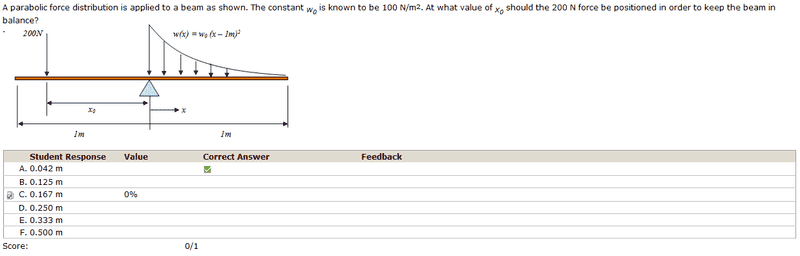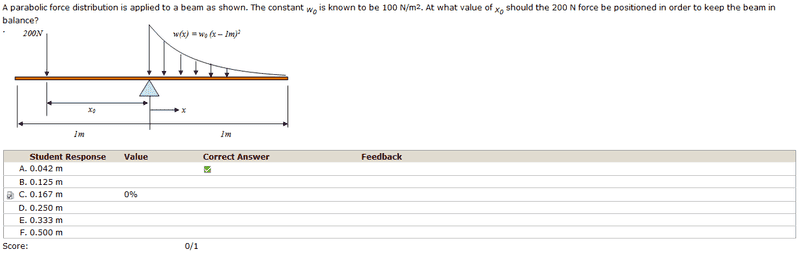# A parabolic force distribution is applied to a beam

## Homework Statement## The Attempt at a Solution

So I noticed the units of w_0 were in N. In other words to find the moment I would have to integrate 100∫01 x2+1-2x dx. However I ended up with the wrong answer.

The correct answer requires that I integrate 100∫01 x2+1-2x x dx

However, if I do it this way I don't see how the units work out... Do you think this is a mistake? I think they wanted w_0 to be a distributed load with units N/m...

HallsofIvy
Homework Helper
The only math involved here is simple integration so I am moving this to the "physic homework" section.

So I noticed the units of w_0 were in N. In other words to find the moment I would have to integrate 100∫01 x2+1-2x dx. However I ended up with the wrong answer.
The units of w_0 are in N/m2. If you integrate 100∫01 x2+1-2x dx, you get force.

The correct answer requires that I integrate 100∫01 x2+1-2x x dx
I suppose you mean 100∫(01 x2+1-2x)x dx. Yes, you need to integrate this to find the moment.

Ray Vickson
Homework Helper
Dearly Missed

## Homework Statement## The Attempt at a Solution

So I noticed the units of w_0 were in N. In other words to find the moment I would have to integrate 100∫01 x2+1-2x dx. However I ended up with the wrong answer.

The correct answer requires that I integrate 100∫01 x2+1-2x x dx

However, if I do it this way I don't see how the units work out... Do you think this is a mistake? I think they wanted w_0 to be a distributed load with units N/m...

In problems of this type it is easier to re-define things a bit, so units do not get in the way. Say the distance is x meters (so x is dimensionless!). Then if the "constant" is w0 N, the constant w0 is also dimensionless. This will make everything work out more easily than the original choice where x is distance (so has attached units of meters) and where w0 is an initial constant with dimensions of N/m2.

Anyway, the point is that you want to match the first moments of the forces, so you want to match force × distance (this is NOT work!) on both sides.

RGV

Thanks everyone! So is w(x) a distributed force? In other words w(x)*dx gives us a small fraction of the force at a certain x and then we multiply this value by x to get the moment?

Yes, w(x) is a distributed loading. As you have pointed out the total force supplied by the loading is the integral of the load function along the length it passes over. This total load is then applied at the centroid of the area underneath the load curve in order to have the same net effect that the distributed load itself had on the beam. From here your problem becomes a simple moment balancing issue.